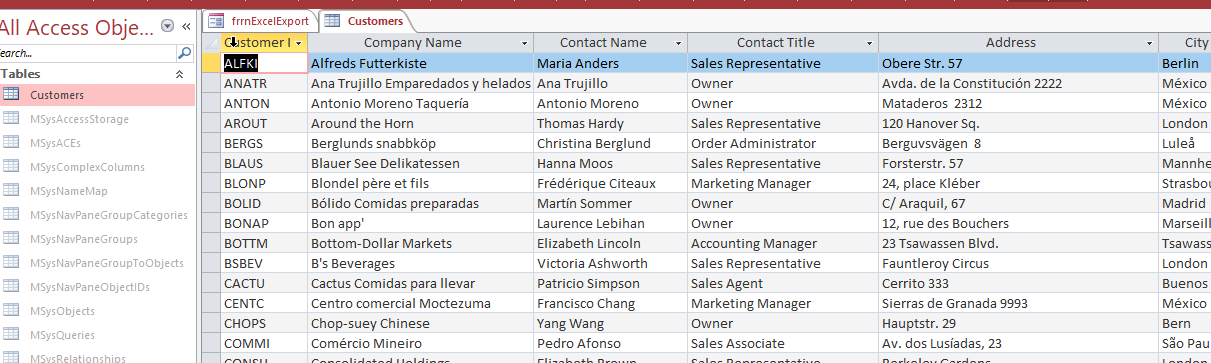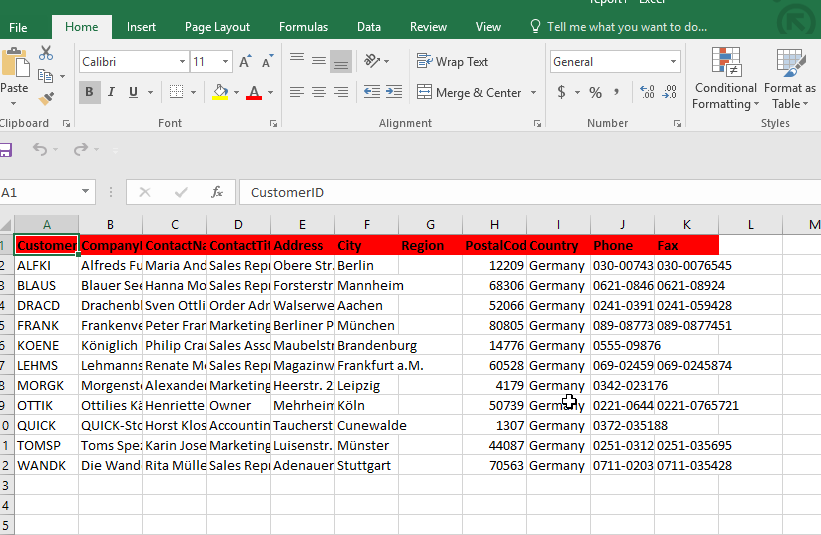# How To Have Access Export A Table To An Excel XLSX In VBA

This was regarding a question I received from this blog:

Hello, I am hoping to find some help. I have an Access DB that I use
to collect data. I have a number of different reporting features. I
would like to add the option for my user to export to excel. When I do
this with a DoCMD code that data exports but now I would like the
ability to format the spreadsheet on export. Is this something that I
can find help with here?

## So it sounds like you want to export a table from access to excel?

Well in this post, I’m going to show you.

In this post we are going to avoid Excel reference complaints by using late binding.

First we’ll have a button which will call our export to Excel function, where we can put any parameters we need:## Here is the code, commented to provide instruction:

```
123456789101112131415161718192021222324252627282930313233343536373839404142434445464748495051525354555657585960616263646566676869707172737475767778798081828384858687888990919293949596979899100101102103104105106107108109110111112113114115116117118119120121122123124125126127128129130131132133134135136137Public Sub GenerateExcelList()

'add a reference to ActiveX Data Objects 2.5 Library in Tools &gt; References

Dim strSQL As String

'Dim objExcelBook As Excel.Workbook
'Dim objExcelSheet As Excel.Worksheet

'USING EARLY BINDING
'Dim objExcelApp As Excel.Application
'Dim objExcelBook As Excel.Workbook
'Dim objExcelSheet As Excel.worksheet

''USING LATE BINDING
Dim objExcelApp As Object
Dim objExcelBook As Object
Dim objExcelSheet As Object

Dim varResultData As Variant
Dim strFields() As String
Dim i As Integer
Dim intCounter As Integer
Dim intCount As Integer

Dim intRST_Fields As Integer
Dim intRST_RecCount As Integer
Dim intRST_StartRow As Integer
Dim intRST_StartCol As Integer

Dim intExcelRow As Integer
Dim intExcelCol As Integer

Dim strData As String

Dim intRecCounter As Integer
Dim strTemplate As String

'TEMPLATE MUST BE SAVED AS TEMPLATE FOR CORRECT FUNCTIONALITY
strTemplate = CurrentProject.Path &amp; "\report.xlsx"

'Set m_objExcelApp = New Excel.Application
On Error Resume Next

strSheet = "Sheet1"

DoCmd.Hourglass True
'-------------------------------------------------------
'STEP 1: EXPORT THE CHART DATA
'-------------------------------------------------------
strSQL = "SELECT * FROM Customers WHERE Country = 'Germany'"

'-------------------------------------------------------------
rst.Open strSQL, CurrentProject.Connection

'don't do all the excel stuff if there is no data...
If Not rst.EOF Then

'this results in an error, not fatal so resume
Set objExcelApp = GetObject(, "Excel.Application")
If objExcelApp Is Nothing Then
Set objExcelApp = CreateObject("Excel.Application")
End If

Set objExcelSheet = objExcelBook.Worksheets(strSheet)

intRST_Fields = rst.Fields.Count
intRST_RecCount = rst.RecordCount
intRST_StartRow = 1

intRST_StartCol = 2

'the recordset data is going to be stored in an array to reduce any object resource consumption
varResultData = rst.GetRows

intRecCounter = 0
intExcelRow = 1
intExcelCol = 1

'make the excel application visible
objExcelApp.Visible = True

'print out all the fields from the recordset.
'the spreadsheet is not zero based, but the recordset is zero based.
For intFieldCount = 1 To rst.Fields.Count
objExcelSheet.cells(intExcelRow, intFieldCount) = rst.Fields(intFieldCount - 1).Name
objExcelSheet.cells(intExcelRow, intFieldCount).Font.Bold = 1
objExcelSheet.cells(intExcelRow, intFieldCount).Font.Name = "Calibri"

objExcelSheet.cells(intExcelRow, intFieldCount).interior.colorindex = 3
Next

'close the recordset because we don't need it anymore
rst.Close
Set rst = Nothing

'now add the column data on the next row
intExcelRow = intExcelRow + 1

'the record set is zero based so if there are 11 rows, we loop from 0 to 10
For intRecCounter = 0 To intRST_RecCount - 1
For intRST_StartCol = 0 To intRST_Fields - 1
'this is how we loop through the rows in our recordset (GetRows) array...
objExcelSheet.cells(intExcelRow, intExcelCol) = varResultData(intRST_StartCol, intRecCounter)
intExcelCol = intExcelCol + 1
Next
'next row, so increment the row destination and reset the column to start at
intExcelRow = intExcelRow + 1
intExcelCol = 1
Next

'CLOSE EXCEL REFERENCES:
Set objExcelSheet = Nothing
Set objExcelBook = Nothing
'objExcelApp.Quit
'Set objExcelApp = Nothing

DoCmd.Hourglass False

Else

rst.Close
Set rst = Nothing

End If
End Sub

```

Here is the end result:#access-vba-export-table-to-excel-xlsx

Let me know if you have questions.

#### “Go Fund Me” Page(\$5 suggested amount)## Free! Subscribe To Our YouTube Channel!